# Modify the circuit of the HX711 module to operate at 3.3V and measure the weight with ESP32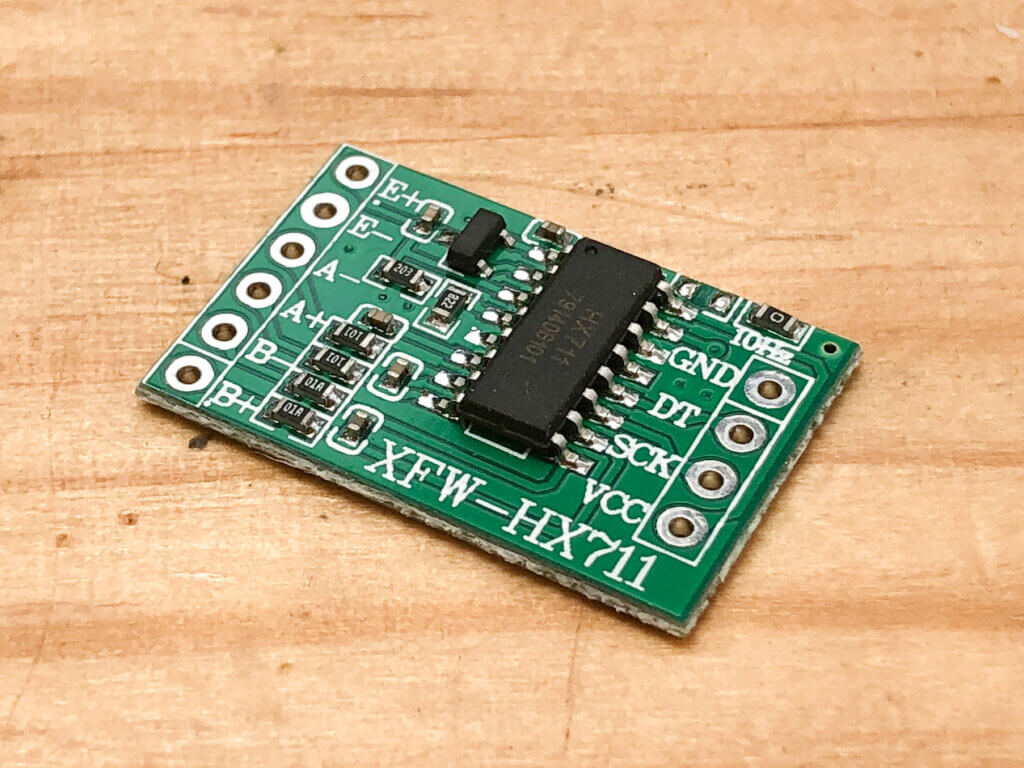## HX711 module is 5V specification

The HX711 module sold on Amazon is designed to work with a 5V power supply. Since the ESP32 has a supply voltage of 3.3V, the HX711 must be operated at 3.3V.

### It really runs at a little lower voltage

The HX711 IC itself can be used in the range of 2.7V to 5.5V according to the datasheet.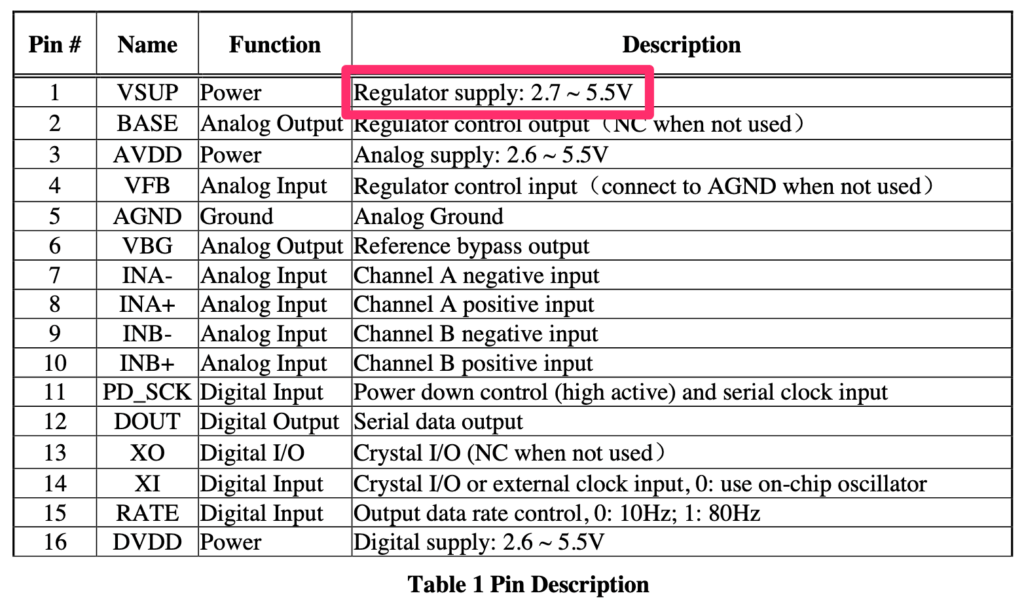Therefore, it can be operated at 3.3V. However, there is a condition. This is the "Power Supply Option" section of the datasheet.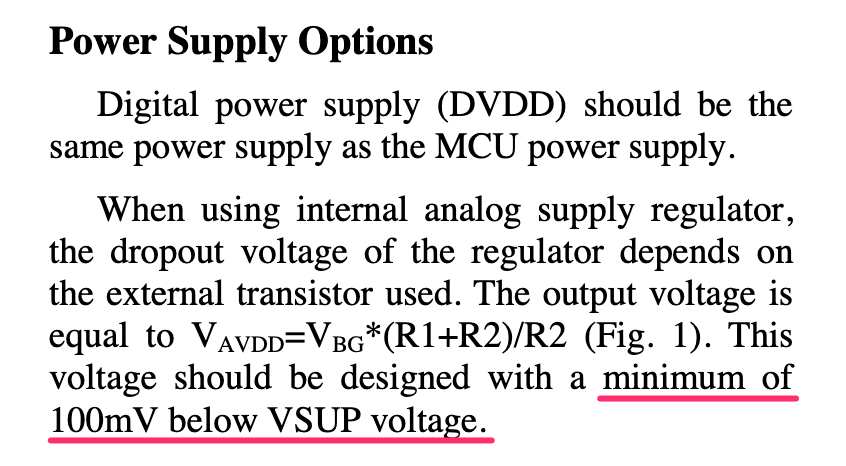Digital power supply (DVDD) should be the same supply voltage as the MCU power supply.
When using internal analog supply regulator, this voltage should be designed with a minimum of 100mV below VSUP voltage.

The HX711 has a built-in regulator for the voltage applied to the strain gages and for the operation of the internal analog circuitry. It means that the voltage generated by that regulator has to be set to at least 100mV lower than the supply voltage. How the voltage of that regulator is determined is as follows

When using internal analog supply regulator, its voltage is
VAVDD = VBG * (R1+R2)/R2

It is written as above. VBG is 1.25V from the datasheet. R1 and R2 read the resistor values mounted on the HX711 module, R1=20kΩ, R2=8.2kΩ. Therefore, the voltage of the regulator of the HX711 module is

VAVDD = 1.25 * ( 20k + 8.2k ) / 8.2k = 4.3V

So, the supply voltage at which the HX711 module operates is at least 4.4V.

Measure the output voltage of the regulator as a test. Measure the voltage between E+ and E- of the module.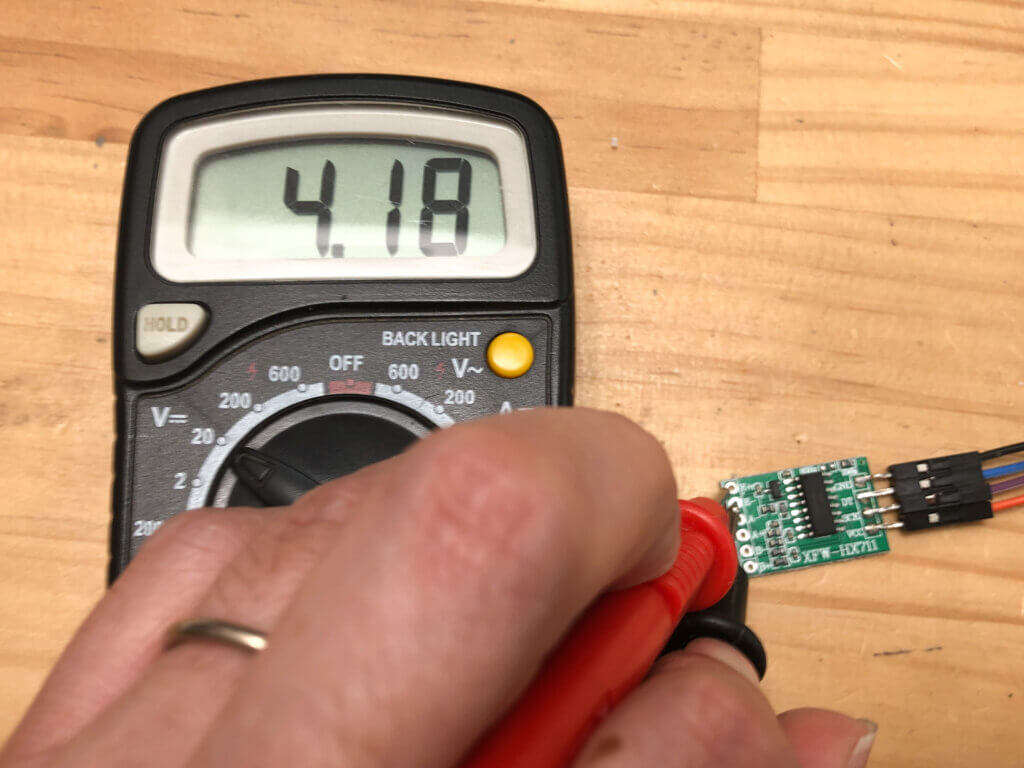The voltage is 4.2V, which is a bit lower than the design, but well within the design.

### Modified to 3.3V specification

To operate with a 3.3V power supply, the voltage of the built-in regulator must be reduced to 3.2V or lower. So, change the resistance value. You can change either R1 or R2, but this time I will change 20kΩ. Add 22kΩ in parallel to 20kΩ to make it 11kΩ. Then.

VAVDD = 1.25 * ( 11k + 8.2k ) / 8.2k = 2.9V

The voltage generated by the regulator will be 2.9V, and the device will work properly even with a 3.3V power supply.

## Modification of HX711 module to 3.3V specification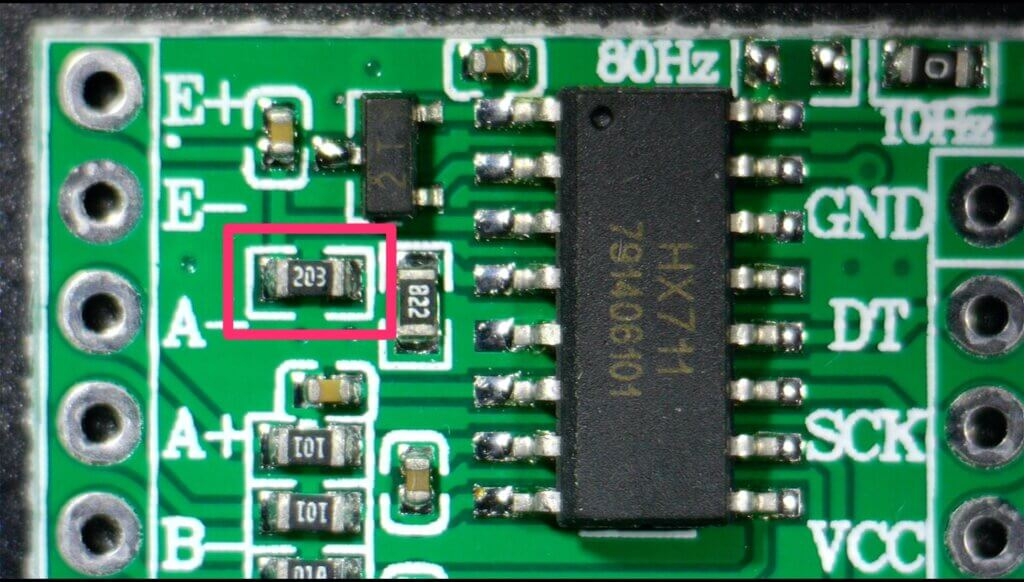Now, let's modify the HX711 module. The part to be modified is the resistor 203 in the upper left corner of the module. Put a 22kΩ chip resistor on the resistor and solder it.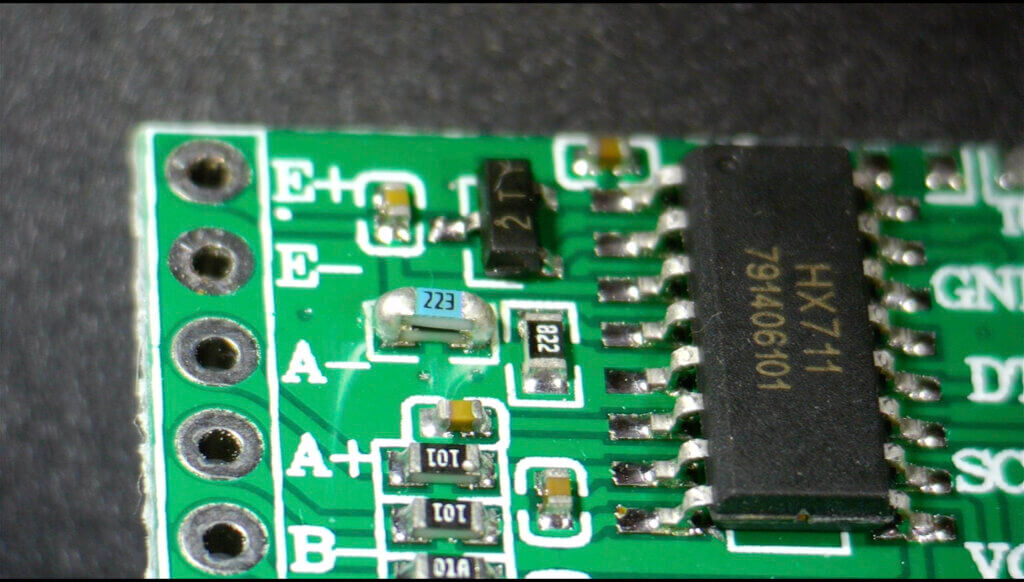Now, 20kΩ and 22kΩ are connected in parallel to be 11kΩ.

Try to measure the voltage of the regulator again.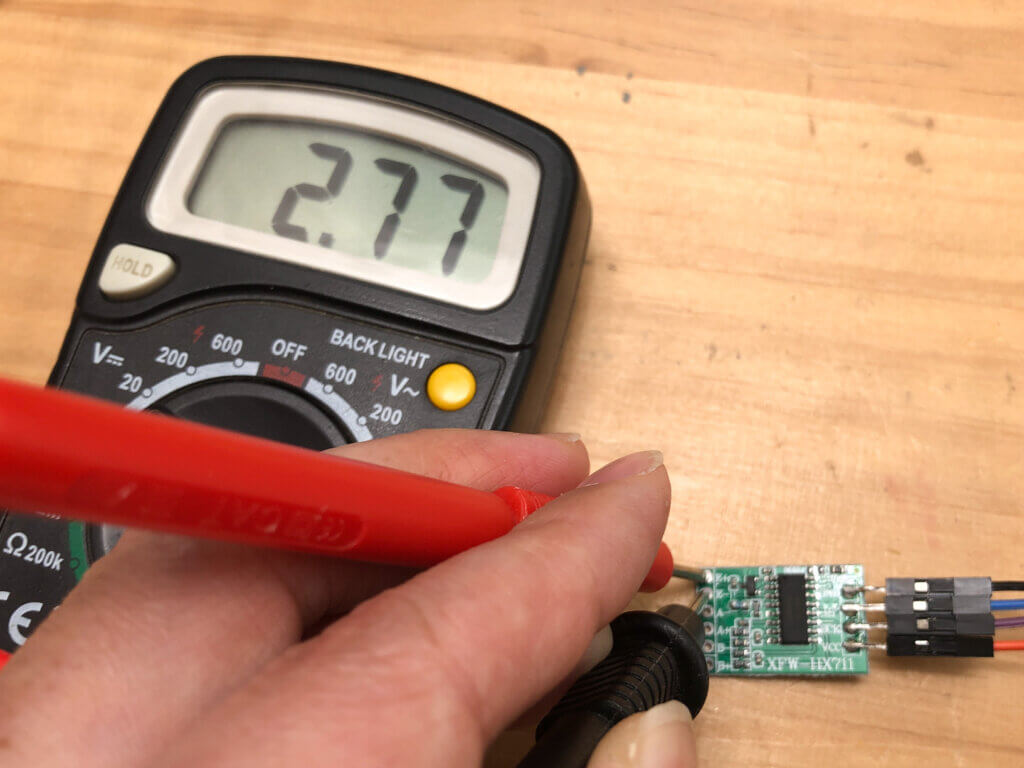The voltage is 2.8V, which is well within the design. Incidentally, the voltage of the regulator was constant at 2.8V whether the supply voltage was set to 5V or 3.3V. The regulator was found to be working properly.

By the way, the voltage of the regulator was only sometimes generated when the HX711 module was just connected to the power supply.

## Connecting to a strain gauge and ESP32

Now let's connect to the ESP32 so that you can get the value of the strain gauge programmatically.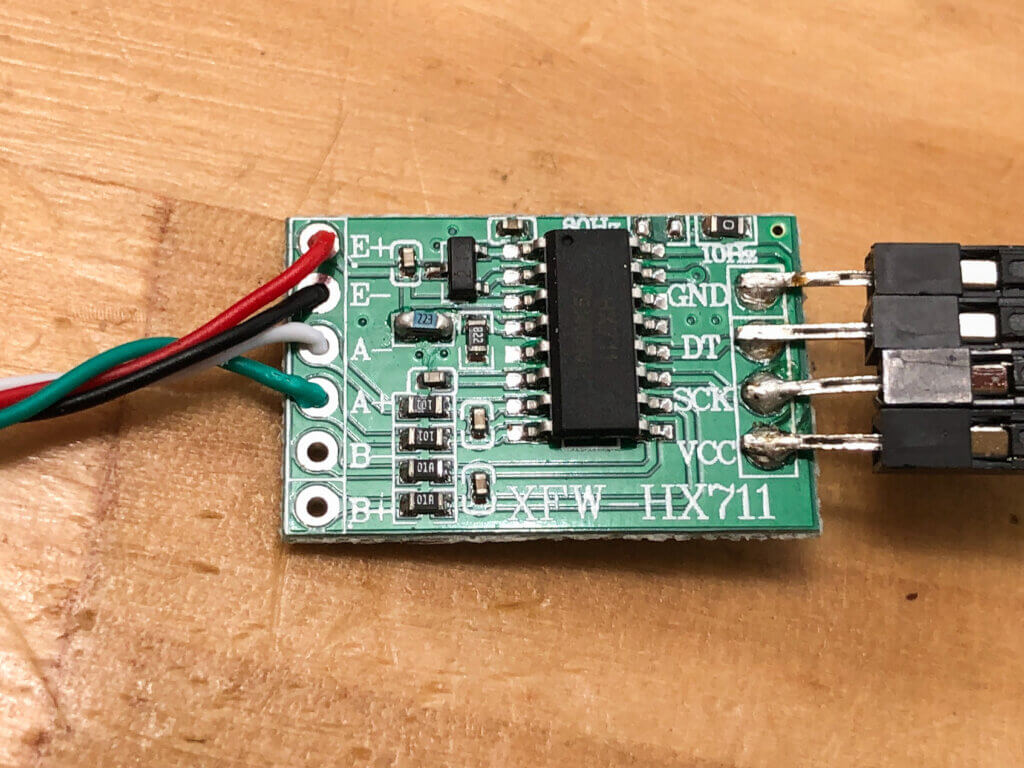The strain gages are connected to the HX711 module as follows.

• E+: Red
• E-: Black
• A-: White
• A+: Green

The strain gauge is attached to the "scale" I made yesterday.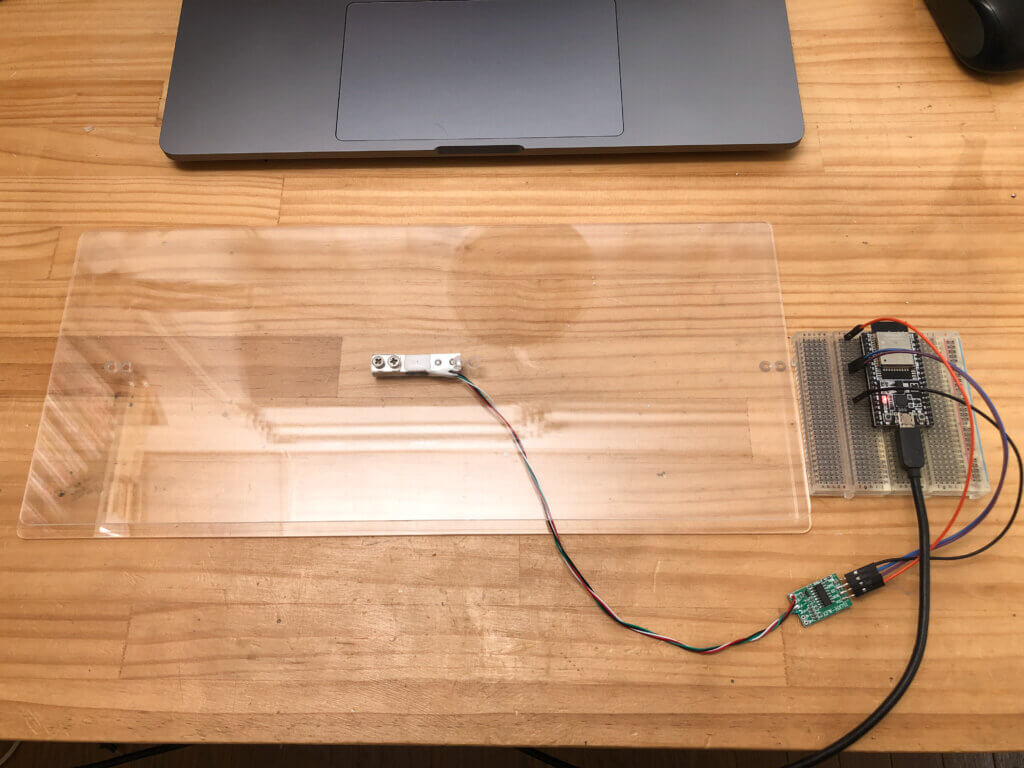Then, the HX711 module is connected to the ESP32 as follows.

• GND：GND
• DT：32
• SCK：33
• VCC：3V3

## Programming the HX711

### Measuring the RAW values first

Make a program with Arduino. The program is as follows.

```#include "HX711.h"
const int DT_PIN = 32;
const int SCK_PIN = 33;
HX711 scale;

void setup() {
Serial.begin(115200);
Serial.println("start");
scale.begin(DT_PIN, SCK_PIN);
}

void loop() {
Serial.println(value);
}
```

It is very simple because it uses the HX711 library. HX711 has a very useful library that is available to the public.

Copy HX711.cpp and HX711.h in the src folder to the folder where you saved the above program.

Now you are ready to go. Compile and write the program to the ESP32.

The measurement results are output to the serial monitor.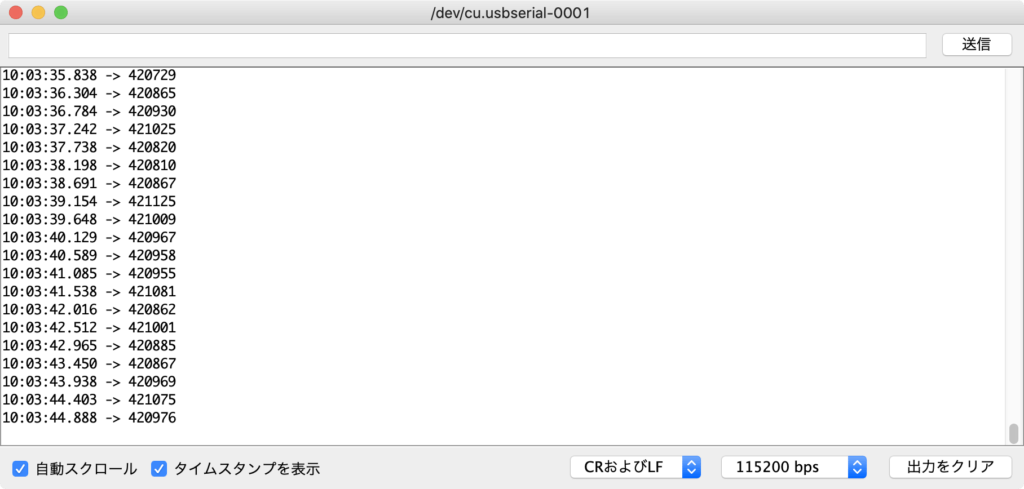The measurement is stable at about 42,000. This value will vary depending on the type of strain gauge and the weight of the scale you have made. The fact that the value is stable is the important point this time, and it means that the measurement is normal.

### Measure the weight

The value obtained earlier is the AD value of the voltage generated by the strain gage being distorted, so it is not yet a weight value in grams. So, from this value, you will convert it to a weight.

Here is the program to convert it to weight.

```#include "HX711.h"
const int DT_PIN = 32;
const int SCK_PIN = 33;
const int SW_PIN = 0;

HX711 scale;
long offset = 0;
double baseWeight = 200.0;

void setup() {
Serial.begin(115200);
Serial.println("start");
scale.begin(DT_PIN, SCK_PIN);
pinMode(SW_PIN, INPUT_PULLUP);
}

int swLowCount = 0;
void loop() {

double weight = (double)(value - offset) * gradient;

if ( digitalRead(SW_PIN) == LOW )
swLowCount++;
else
{
//short push(2sec) and release is enter the Offset value measuring mode
if ( swLowCount > 0 && swLowCount < 4) {
Serial.print("Offset value measuring...");
Serial.printf(" Done. Offset value = %ld\n", offset);
}
//long push(6sec) and release is enter the Gradient value measuring mode
if ( swLowCount > 6 )
{
baseValue -= offset;
}

swLowCount = 0;
}
}
```

To convert the values obtained from HX711 to weights, the following steps are required.

1. Subtract the value of nothing (remove offset)
2. Know the amount of change when an object of known weight is put on it (calculate the proportionality coefficient)

#### 1. Offset removal

The strain gauge is already distorted by the weight of itself and the scale's weight. Therefore, even if you don't put anything on the scale, you will get some constant value.

Thus, this value (offset) must always be subtracted from the AD value.Run the program, first making sure that nothing is put on the scale.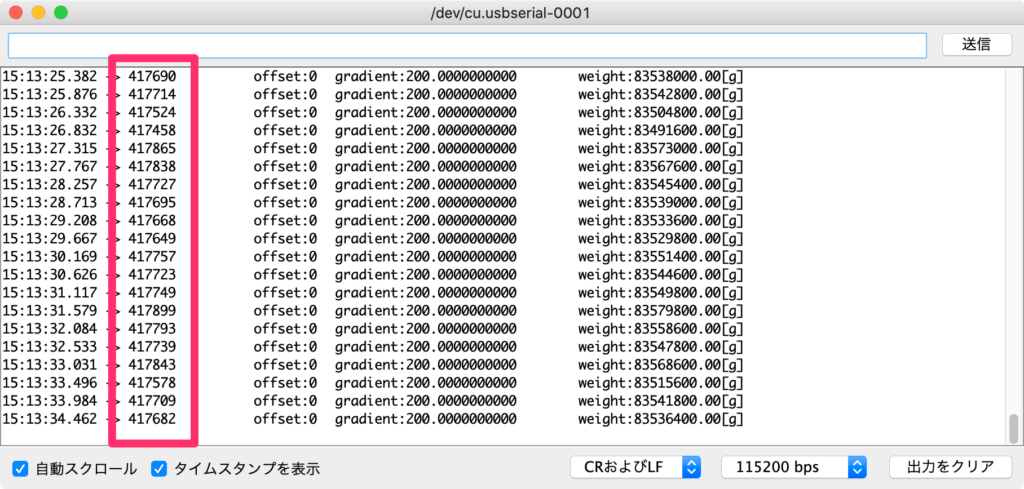The value is roughly 418,000. This is the offset.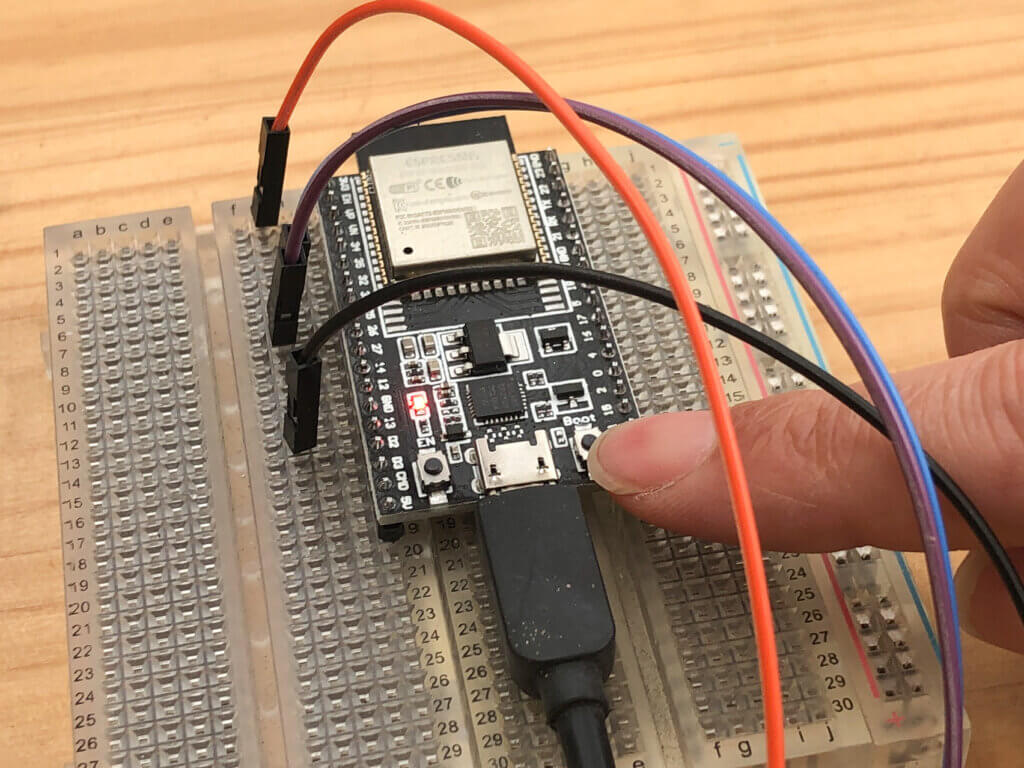Press and hold the ESP32 Boot button (IO0) for a few seconds and then release it.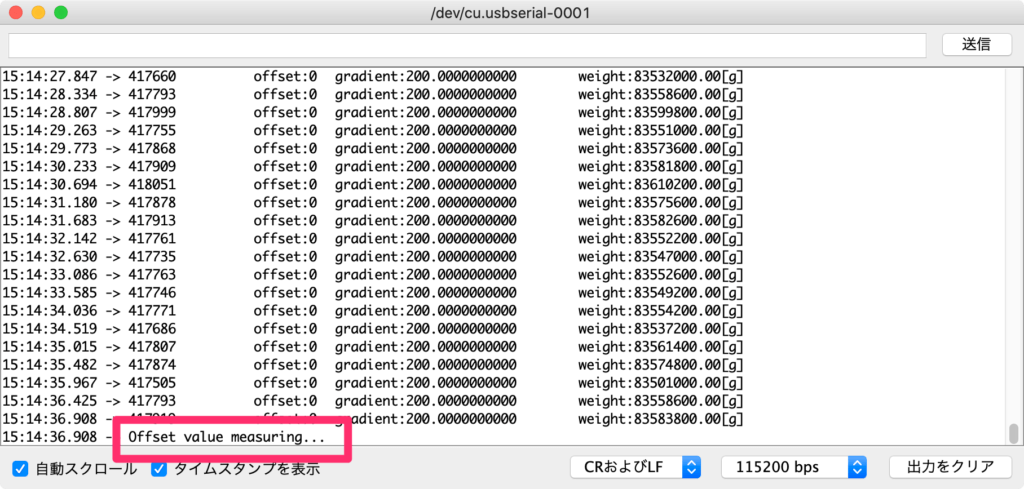Enter the offset measurement mode.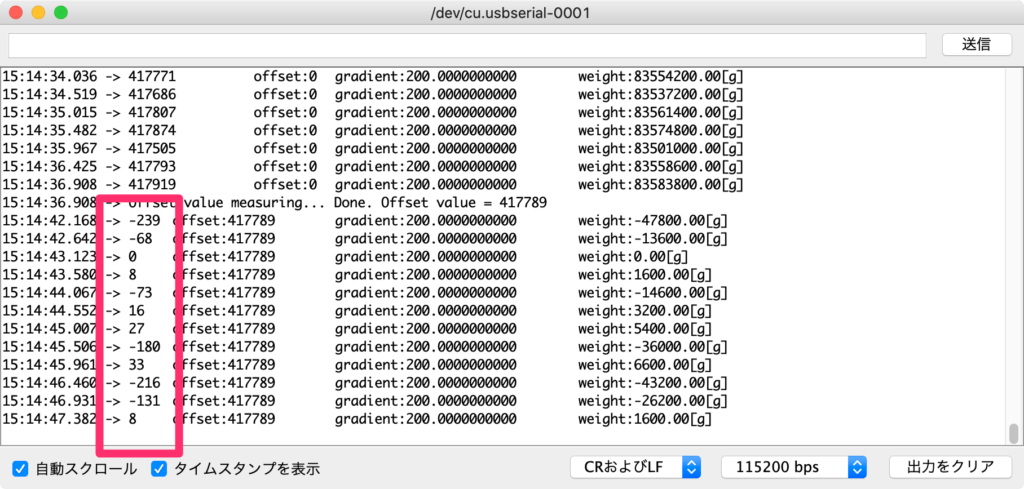The offset will be measured for about 5 seconds and then the offset will be removed from the AD value.

Now the scale's weight will be deducted.

#### 2. Calculate the proportionality coefficient

The value with the offset subtracted is still not the weight value. It is an AD value that is 0 when nothing is put on the scale. So, calculate the proportionality factor to convert the AD value to weight.

The AD value increases in proportion to the weight. Therefore, if we know how many grams it is at one AD value, can convert it to weight by multiplying it by the measured AD value.

Specifically, put an object with a known weight, say 200g, and check how many AD values it has. Then, by doing the 200/AD value, calculate the proportionality factor for converting to weight.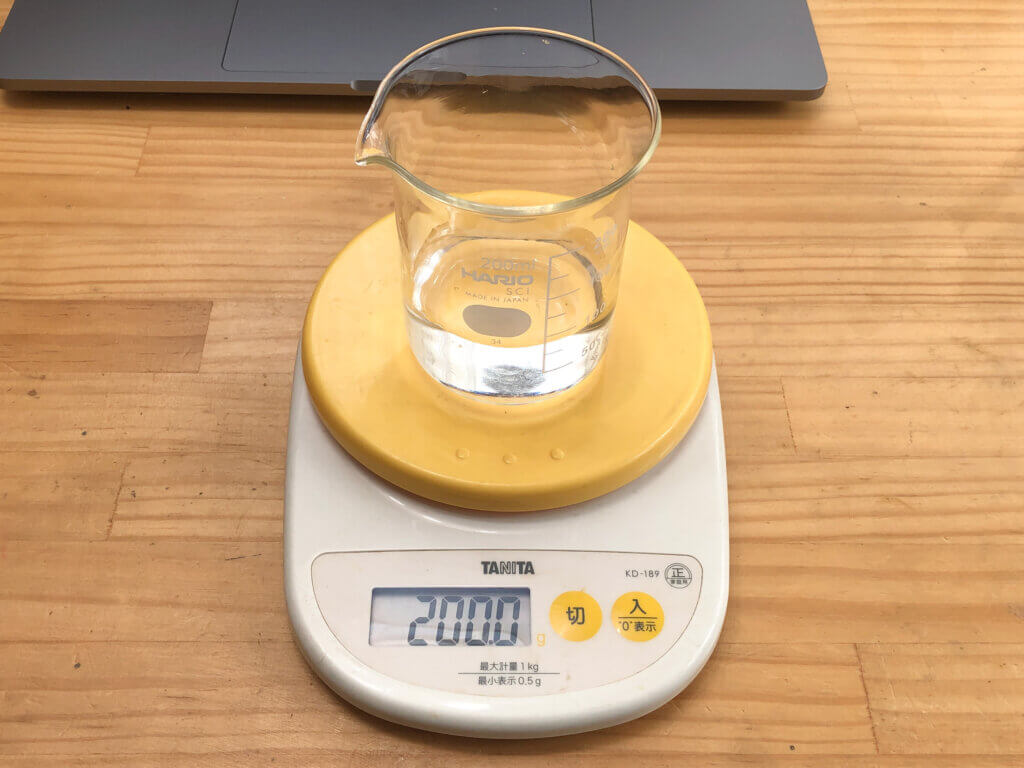Prepare a 200g weight by filling a container with water.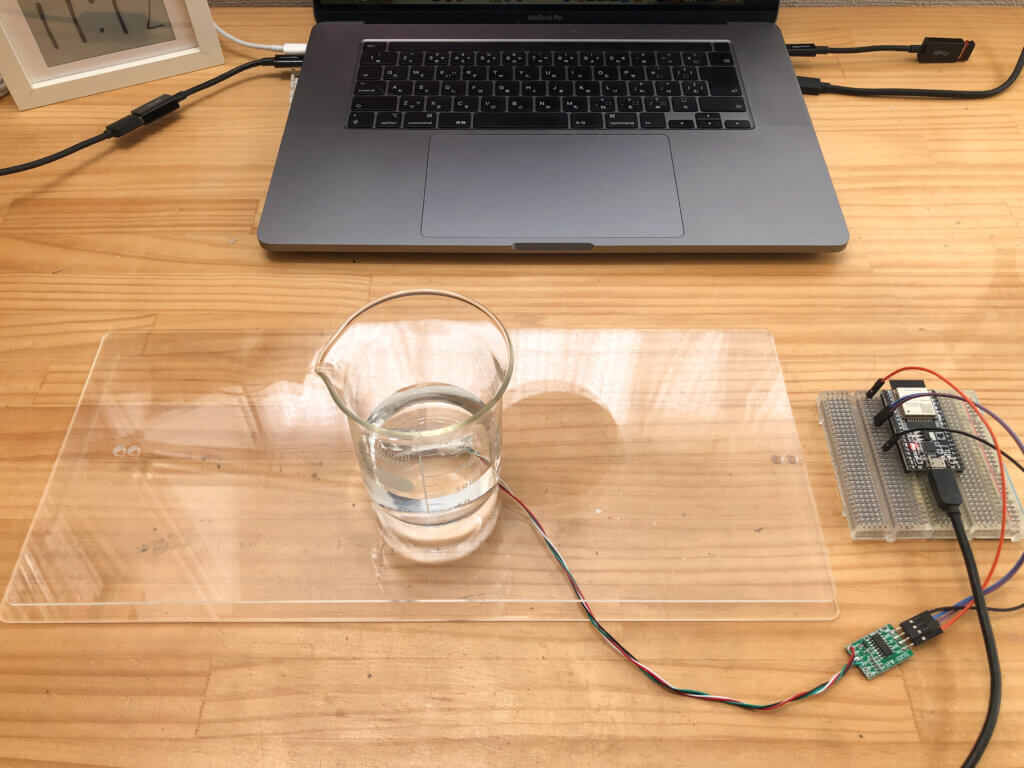Put it on the scale.Press and hold the ESP32 Boot button (IO0) for more than 6 seconds and release it.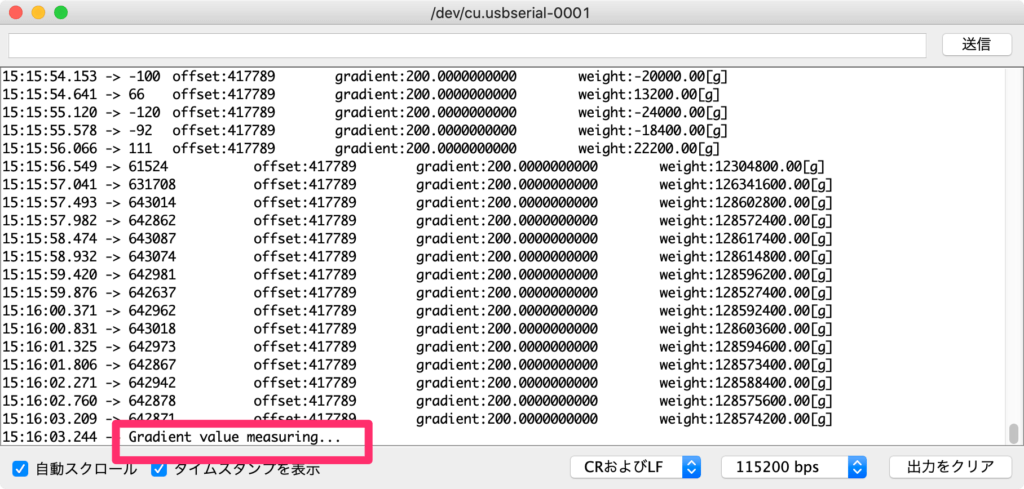Enter the calculate proportionality coefficient mode.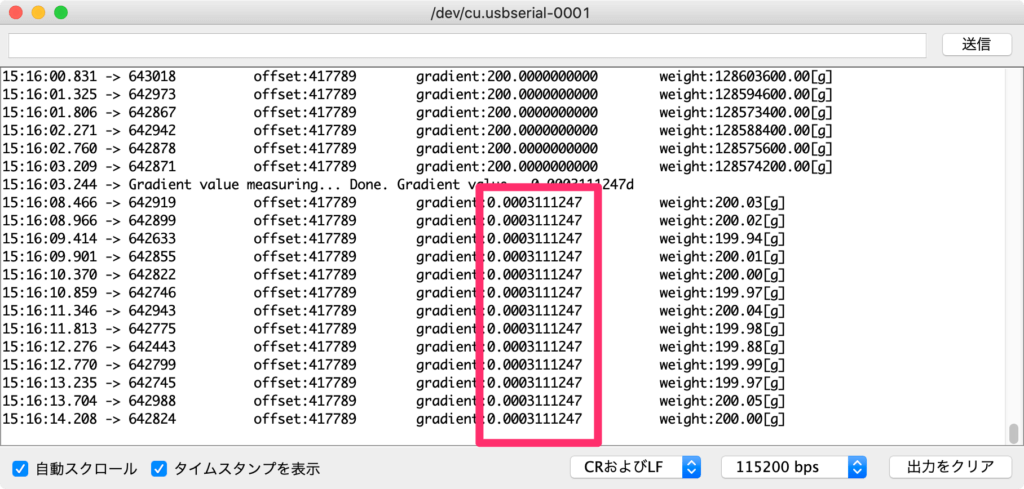The proportionality factor will be measured in about 5 seconds. The proportionality coefficient is 0.000311...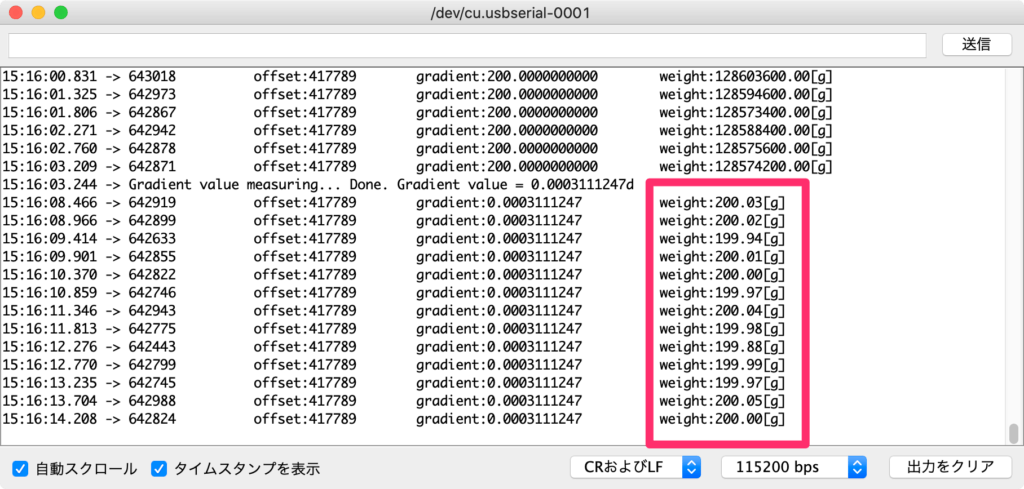The calculated proportionality factor will now be used to convert the AD value to weight. Since this is 200g of water, it also weighs 200g.

It is now a "scale" that can be used to measure weight.

If you want to calibrate at 100g instead of 200g, then set line 9 of the program to 100.0.

If you set the offset value and the proportionality coefficient value obtained in this process to the offset in line 7 and the gradient in line 8 of the program beforehand, you will be able to measure the weight from the beginning even after resetting the program.